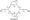# Control Systems July 2009

Note:

1. Answer any FIVE full questions, choosing at least two questions from each.

2.   Missing data may be suitably assumed

Part-A

1    a. Mention the merits and demerits of open loop and closed loop control systems and give an example for each.

b.   For the mechanical system shown in figure Q1 (b), obtain the force-voltage analogous network.

c.   Obtain the transfer function of an armature controlled dc servomotor.

2 a. Explain briefly the following terms:

i)      Forward path.         ii) Path gain.             iii) Loop gain.           iv) Canonical form.

b Obtain the C/R ratio for the block diagram shown using block-diagram reduction technique.c. Find C(s) R(s) by Mason’s gain formula (Fig. Q2 (c)).3 (a)Derive an expression for the under damped response of a second order feed back control system for step input.

(b)Obtain expressions for rise-time and peak-time for a second-order feed back system response for a step input (under-damped case).

(C) A positional servomechanism is characterized by an open loop transfer functionG(s)=, where k and a are positive constants, for a unity feedback. Find the valuess(s+a) of k and a for a damping coefficient value of 0.6 and damped frequency of 8 rad/sec. Also find the peak value of the response when the system is excited by a step of 2 volts.

4 (a)Find KP, Kv and Ka for the system whose open loop transfer function is given by, G(s)H(s)= 1Q(S+2XS+3) s(s+l)(s+5)(s+4) Also find the steady-state error for an input r(t)=3 +1 +1.

(b)Explain Routh-Hurwitz ‘s’ criterion for determining the stability of a system and mention by limitations. A unity feedback control system has: k(s+13) G(s)=s(s+3)(s+7) Using the Routh’s criterion, calculate the range of £k’ for which the system is

i)    Stable

ii)   Has its closed loop poles more negative than – 1.

Part-B

5 (a)Explain briefly the following terms with respect to root-locus technique:

i)    Centroid.

ii)   Asymptote.

ii) Break away point.

(b) Sketch the root locus plot for a closed loop system having an open-loop transfer function: k(s+2)G(s)H(s)=for all values of k from 0 to oo. Comment on the stability of the system. s(s+l) Show that a part of the root-locus for the open loop transfer function: k(s+2)

G(s)H(s )=is a circle. s(s+l)

6 (a)The open loop transfer function of a negative feedback control system is: GH(s)=rr-s(s+l)(s+X) Sketch the polar plot and hence find the following:

i)       Phase cross-over frequency.

ii)      Gain cross-over frequency.

iii)     Gain-margin.

iv)     Phase-margin.

(b) Explain in detail the procedural steps of Nyquist stability criterion.

(c) A feed back control system has loop transfer function: GH(s)=s(s+l) Sketch the SyQuest plot and comment on the stability of a system.

7 a. Derive expressions for resonant peak and resonant frequency for a second order system.

b.  Find the open-loop transfer function for a unity feed back second order control system for which resonant peak is 1.1 units and resonant frequency is 11.2 radians/sec. (06 Marks)

c.  Sketch the Bode-piot for the transfer function: G(s)=Ks2 (l+0.2s)(l+0.02s) Determine the value of ‘k’ for the gain cross over frequency to be 5 rad/sec.

8 a. Compare transfer function approach and state variable approach of analyzing control system.

b. A feed back system is characterized by the closed loop transfer function:

GH(s)=- s2 +3s+3 s3+2s2+3s+l Obtain its state model.

c.   State the properties of state transition matrix. Obtain the state transition matrix for: